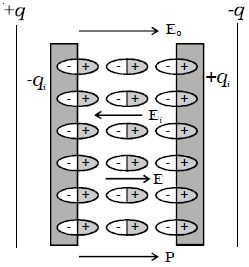Physics

# What is Polarization of Dielectric Material?

Polarization of Dielectric Material: Consider a parallel plate capacitor with +q and –q charges.Fig: Polarization of dielectric material

Let E0 be the electric field between the plates in the air. If a dielectric slab is introduced in the space between them, the dielectric slab gets polarized. Suppose +qi and –q1 be the induced surface charges on the face of dielectric opposite to the plates of the capacitor (Figure). These induced charges produce their own field Ei which opposes the electric field E0. So, the resultant field, E < E0. But the direction of E is in the direction of E0.

so, E = E0 + (–Ei)

(Here E1 is opposite to the direction of E0)